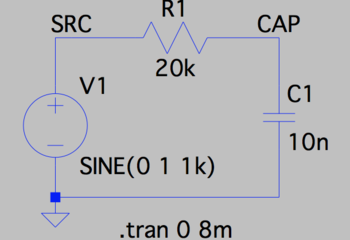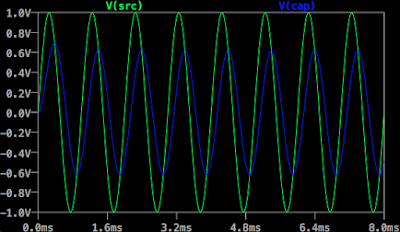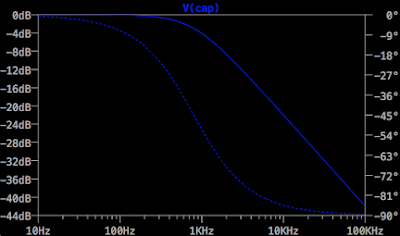## Saturday, December 12, 2020

### Audio Amplifier Feedback - Amplifier with Multiple Poles Inside Feedback Loop

This post is a part of the series on audio amplifier feedback. The contents of the series can be found here.

In the last post, I mentioned that any real amplifier's gain sooner or later goes down as the frequency goes up. As the gain declines, the phase at the output starts lagging that at the input (the amplifier has a pole):Declining gain and lagging phase as the frequency grows are the characteristics of a low-pass filter:With some simplification, one can say that every capacitance in a circuit can form a low pass filter and can create a pole. Even in a very simple circuit, there are many capacitances, intended and parasitic:
so there are many poles.
Let's say an amplifier has three poles, at 2kHz, 1MHz and 20MHz, and is placed inside a feedback loop with B=-1/10 (say a resistive divider made of a 9kOhm and a 1kOhm resistors from the output of the amplifier to its inverting input):
The Bode plot of the amplifier's open loop gain and the ideal closed loop gain:
If we apply a square wave to the input of such an amplifier, at the output we will observe the following:

which is not what was intended. The signal transfer function has a pronounced peak and is not the flat 20dB line that the feedback network demands:

Turns out that at about 4.5MHz, where the open goop gain $Aol$ approaches $1/B$and the loop gain $Aol \times B$ approaches unity, the amplifier's multiple poles together create a phase lag of almost 180 degrees. Together with the 180 degrees created by applying the feedback to the inverting input, the phase shift is close to zero at the point where the loop gain is unity. As a result, the signal transfer function $STF={LG \over {1 - LG}}{1 \over B}$ and the error transfer function $ETF={1 \over {1 - LG}}$ can become very large, leading to instability.
To avoid instability and make $STF$ and $ETF$ what they should be, the amplifier needs to be compensated, which will be the topic of my future posts.